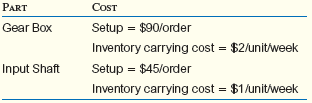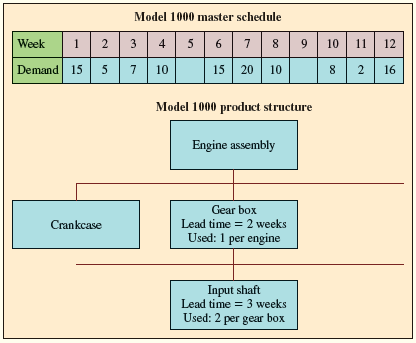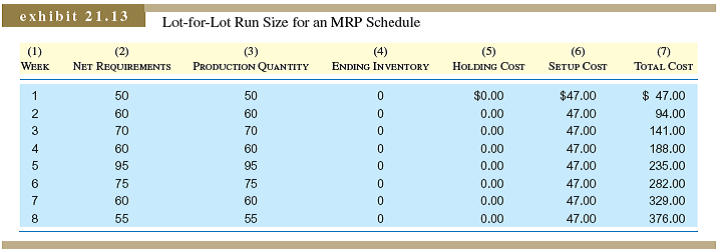# [Solved] Phil Would Like to Consider the Costs That His Accountants

## Phil would like to consider the costs that his accountants are currently using for inventory carrying and setup for the gear boxes and input shafts. These costs are as follows:Given the cost structure, evaluate the cost of the schedule from question 1. Assume inventory is valued at the end of each week.Question 1 for Reference:Initially, assume that Phil wants to minimize his inventory requirements. Assume that each order will be only for what is required for a single period. Using the following forms, calculate the net requirements and planned order releases for the gear boxes and input shafts. Assume that lot sizing is done using lot-for-lot (L4L).Lot-for-Lot for Reference:Tags

10+ million students use Quizplus to study and prepare for their homework, quizzes and exams through 20m+ questions in 300k quizzes.

Explore our library and get Decision Science Homework Help with various study sets and a huge amount of quizzes and questions

### Related Questions

Get Free Access Now!